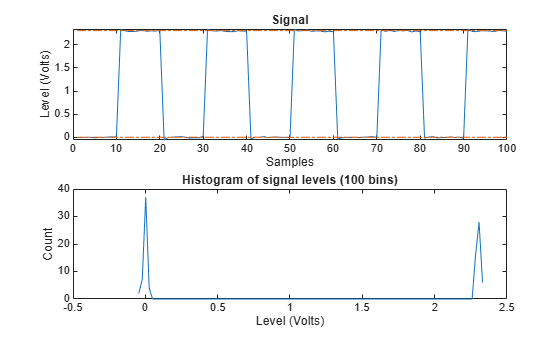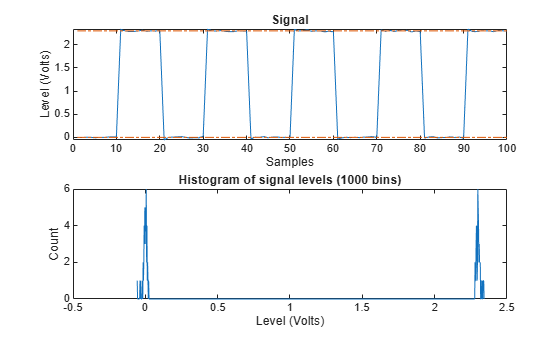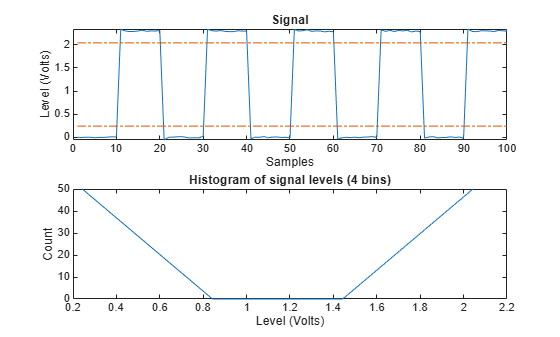Documentation

# statelevels

State-level estimation for bilevel waveform with histogram method

## Syntax

``LEVELS = statelevels(X)``
``LEVELS = statelevels(X,NBINS)``
``LEVELS = statelevels(X,NBINS,METHOD)``
``````[LEVELS,HISTOGRAM] = statelevels(___)``````
``````[LEVELS,HISTOGRAM,BINLEVELS] = statelevels(___)``````
``statelevels(___)``

## Description

````LEVELS = statelevels(X)` estimates the low and high state levels in the bilevel waveform `X` using the histogram method. See Algorithms.```
````LEVELS = statelevels(X,NBINS)` specifies the number of bins to use in the histogram as a positive scalar. If unspecified, `NBINS` defaults to `100`.```
````LEVELS = statelevels(X,NBINS,METHOD)` estimates state levels using the mean or mode of the subhistograms. Valid entries for `METHOD` are `'mean'` and `'mode'`. `METHOD` defaults to `'mode'`.```
``````[LEVELS,HISTOGRAM] = statelevels(___)``` returns the histogram of the values in `X`.```
``````[LEVELS,HISTOGRAM,BINLEVELS] = statelevels(___)``` returns the centers of the histogram bins.```
````statelevels(___)` displays a plot of the signal and the corresponding histogram.```

## Examples

collapse all

Estimate the low- and high-state levels of 2.3 V underdamped clock data. Plot the data with the estimated state levels and subhistograms.

```load('clockex.mat','x') statelevels(x)``````ans = 1×2 0.0027 2.3068 ```

Estimate the low- and high-state levels of 2.3 V underdamped clock data sampled at 4 MHz.

Use the default number of bins and modes of the subhistograms to estimate the state levels.

```load('clockex.mat','x','t') levs = statelevels(x)```
```levs = 1×2 0.0027 2.3068 ```

Plot the clock data with the lines indicating the estimated low- and high-state levels.

`statelevels(x)````ans = 1×2 0.0027 2.3068 ```

Estimate the low- and high-state levels of 2.3 V underdamped clock data sampled at 4 MHz.

Use the default number of bins and means of the subhistograms to estimate the state levels. Plot the clock data with the lines indicating the estimated low- and high-state levels.

```load('clockex.mat','x','t') statelevels(x,1e3,'mean')``````ans = 1×2 -0.0014 2.3014 ```

Estimate the low- and high-state levels of 2.3 V underdamped clock data sampled at 4 MHz. Return the histogram counts and histogram bin centers used in the histogram method. Use four bins.

```load('clockex.mat','x','t') [levs,histog,bilevs] = statelevels(x,4)```
```levs = 1×2 0.2427 2.0428 ```
```histog = 4×1 50 0 0 50 ```
```bilevs = 4×1 0.2427 0.8427 1.4428 2.0428 ```

Plot the waveform and annotate the levels.

`statelevels(x,4)````ans = 1×2 0.2427 2.0428 ```

## Input Arguments

collapse all

Bilevel waveform, specified as a real-valued vector.

Number of histogram bins, specified as a real positive scalar.

State-level estimation method in the subhistograms, specified as `'mode'` or `'mean'`. `METHOD` specifies the statistic to use for the estimation of the low- and high-state levels. See Algorithms.

## Output Arguments

collapse all

Levels of low and high states, returned as a two-element positive row vector. The vector of state levels is estimated by the histogram method. The first element of `LEVELS` is the low-state level and the second element is the high-state level.

Histogram counts, returned as a column vector with `NBINS` elements containing the number of data values in each histogram bin.

Histogram bin centers, returned as a column vector. The column vectors contain the bin centers for the histogram counts in `HISTOGRAM`

collapse all

### State

A state is a particular level, which can be associated with an upper- and lower-state boundary. States are ordered from the most negative to the most positive. In a bilevel waveform, the most negative state is the low state. The most positive state is the high state.

### State-Level Tolerances

Each state level can have associated lower- and upper-state boundaries. These state boundaries are defined as the state level plus or minus a scalar multiple of the difference between the high state and the low state. To provide a useful tolerance region, the scalar is typically a small number such as 2/100 or 3/100. In general, theregion for the low state is defined as

``

whereis the low-state level andis the high-state level. Replace the first term in the equation withto obtain thetolerance region for the high state.

The following figure illustrates lower and upper 2% state boundaries (tolerance regions) for a positive-polarity bilevel waveform. The red dashed lines indicate the estimated state levels.## Algorithms

`statelevels` uses the histogram method to estimate the states of a bilevel waveform. The histogram method is described in . The steps of this method are:

1. Determine the maximum and minimum amplitudes and amplitude range of the data.

2. For the specified number of histogram bins, determine the bin width, which is the ratio of the amplitude range to the number of bins.

3. Sort the data values into the histogram bins.

4. Identify the lowest-indexed histogram bin, ${i}_{\text{low}}$, and highest-indexed histogram bin, ihigh, with nonzero counts.

5. Divide the histogram into two subhistograms:

The indices of the lower histogram bins are ${i}_{\text{low}}\le i\le \frac{1}{2}\left({i}_{\text{high}}-{i}_{\text{low}}\right)$.

The indices of the upper histogram bins are ${i}_{\text{low}}+\frac{1}{2}\left({i}_{\text{high}}-{i}_{\text{low}}\right)\le i\le {i}_{\text{high}}$.

6. Compute the state levels by determining the mode or mean of the lower and upper histograms.

## References

 IEEE® Standard on Transitions, Pulses, and Related Waveforms, IEEE Standard 181, 2003, pp. 15–17.